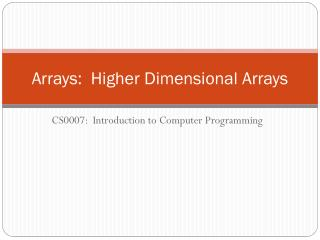Download PresentationArrays: Higher Dimensional Arrays

# Arrays: Higher Dimensional Arrays

Download Presentation## Arrays: Higher Dimensional Arrays

- - - - - - - - - - - - - - - - - - - - - - - - - - - E N D - - - - - - - - - - - - - - - - - - - - - - - - - - -
##### Presentation Transcript

1. Arrays: Higher Dimensional Arrays CS0007: Introduction to Computer Programming

2. Review • If the == operator has two array variable operands, what is being compared? • The reference variables held in the variables. • How do you compare two arrays to see if they hold the same elements? • Iterate through the arrays and compare them element by element. • How are the sum and average array algorithms similar? • Both iterate through the array and add each element to an accumulator variable. • How are the minimum and maximum element algorithms similar? • The current minimum or maximum element is compared to each element, if an element is smaller (minimum case) or larger (maximum case) than the current minimum or maximum, the element replaces it. • If you intend to use a partially filled array, what information must be kept in addition to the array itself? • The current number of elements in the array • How does the Sequential Search Algorithm work? • Look at every element in the array starting at the first element and check if it is the target value.

3. Two Dimensional Arrays • Imagine now we want to keep 10 test scores for 30 students. • How would we represent this in a program with what we’ve learned? • Answer: You would need either 30 10-element arrays or 10 30-element arrays. • This was the problem arrays were supposed to solve! • What we have been working with so far are considered one dimensional arrays, because they only extend along one dimension (one set of data). • Java also allows for higher dimensional arrays, for example, two dimensional arrays. • Two dimensional arrays can be though of having a table of rows and columns in memory. • So, a better solution to the above question is what? • Answer: Have a 30 x 10 or a 10 x 30 two dimensional array!

4. Two Dimensional Array Example • New Topics: • Two Dimensional Arrays

5. Two Dimensional Arrays • Declaring a two dimensional array is very similar to declaring a one dimensional array. • For example, to declare an integer array variable with 3 rows and 4 columns called numbers you would: int[][] numbers = newint; • The two sets of brackets on the left side of the assignment operator indicates the variable is a reference variable to a two dimensional array. • The  on the right side indicates the array object will have 3 rows. • The on the right side indicates the array object will have 4 columns. • Each array element has two indices, one indicating the row and one the column. • For example, to access the element in the first row and third column of the above declared numbers array you would: numbers

6. Two Dimensional Arrays • To initialize a two dimensional array you encapsulate each row in curly brackets, separating elements by commas. You separate each row by commas and put the entire array in curly brackets: int[][] numbers = {{1,2,3}, {4,5,6}, {7,8,9}}; or… int[][] numbers = {{1,2,3}, {4,5,6}, {7,8,9}}; • Another way to think of two dimensional arrays is that a two dimensional is a one dimensional array of one dimensional arrays. • Said another way, it is a reference variable to an array that holds reference variables to arrays. • This is apparent when using the length data member in two dimensional arrays: int[][] numbers = newint; numbers.length – the number of rows numbers.length– the number of columns in the first row • Example: TwoDimLengths.java

7. Class Programming Practice: ClassScores.java • Create a program where a user can enter 3 students scores on 3 quizzes. • Then, the program should tell the user the average for each student, each quiz, and overall class average for all quizzes.

8. Ragged Arrays • Since the rows of a two dimensional array are arrays themselves, they can have different lengths: • When the rows of a two dimensional array are of different lengths, the array is known as a ragged array. int[][] ragged = newint[]; ragged = newint; ragged = newint; ragged = newint; ragged = newint; • Example: RaggedArray.java

9. Higher Dimensional Arrays • Java does not impose a limit on how many dimensions an array can be. • For instance we can create a three dimensional array: int[][][] numbers = newint; • You can have higher dimensional arrays by simply defining the size of the dimension. • You can also have ragged arrays of higher dimensions. • For instance you can have a three dimensional array with 3 two-dimensional arrays of sizes 3x3, 4x5, and 2x6.